# Continuous shouldered well model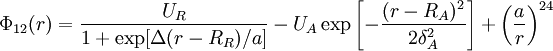$\Phi_{12}(r) = \frac{U_R}{1 + \exp [\Delta(r-R_R)/a]} -U_A \exp \left[ -\frac{(r-R_A)^2}{2\delta_A^2} \right] + \left(\frac{a}{r}\right)^{24}$
where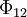$\Phi_{12}$ is the intermolecular pair potential,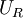$U_R$ is the energy of the repulsive shoulder,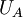$U_A$ is the energy of the attractive well,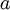$a$ is the diameter of the particles, and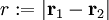$r := |\mathbf{r}_1 - \mathbf{r}_2|$.